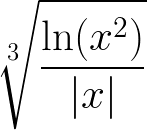# Introduction to Programming

## Public Resources

If provided, read carefully additional texts that can contain some helpful information on the origins of the files, licence agreements, etc.

### Lesson 1

• subject conditions
• recommended IDE (Visual Studio Code)
• template project in C++

### LESSON 2

Prerequisites

• variables, basic data types
• numeric, logic, and bitwise operators
• basic loops
• standard input/output with defined format
• functions

• practice the above mentioned items
• read some numeric data from the standard input
• write a variable of unsigned int data type to the standard output in a binary form
• create a new method that will print a given number in its binary form
• ask the user for a number, read it and print it in the normal, binary and hexadecimal form

One-minute code

• Four easy examples that demonstrate how to repeatedly prompt the user to enter a number. Try to find the differences. -> DOWNLOAD

### LESSON 3

Prerequisites

• functions/methods ... basic notations, return values, etc.
• random numbers
• arrays

• make a static declaration of an array of ten elements and initialize such array to following values {5, 7, 8, 6, 20, 100, 15, 13, 4, 5}
• create a function that fills a given array with random numbers from interval <0,1>
• create a function that returns the largest number in a given array and index of this number
• create an array of 1M elements
• create a matrix M[m x n]. Fill the matrix with integer numbers such that the M[i, j] = i + j, where i∈<0, m-1>, j∈<0,n-1>, m=100, and n=100.
• create a matrix M[1000x1000]. Fill the matrix with random numbers from interval <0,255>. This matrix represents a grayscale image. Create a histogram for this image.

### Lesson 4

Prerequisites

• functions
• standard math library

• a short recap of the previous tasks
• write a function f(x) that returns a single value for every x from the interval <-1000,1000>, if (x mod 3 == 0). The function is given by the following formula:• think about the optimization. Do you really need the division and modulo? What about the absolute value?
• try to modify the function such that the user can set the interval (upper and lower bound).

### Lesson 5

Prerequisites

• arrays, multi-dimensional arrays
• functions

• create two vectors of a given dimension and fill them with random values from the interval <0,1>
• write a function that returns a dot product of two vectors
• let consider the first vector to be a column vector (a matrix of dimension [Mx1]) and create a function that multiplies this column vector with a row vector (a matrix of dimension [1xM]).

Prerequisites

• structures
• functions

### LESSON 7

Prerequisites

• structures
• arrays, multi-dimensional arrays
• functions

Topics and Tasks - WINTER SKY

• create an empty project and copy this code of helper functions
• create a structure that will represent a Snowflake in 2D space (x,y)
• create a 2D array that will represent a sky and initialize the array to empty values (e.g. ' ')
• initialize N snowflakes with random positions (think about XY vs. YX coordinates)
• create methods, that remove snowflakes from the sky, move with snowflakes, and set snowflakes to the sky
• print the sky in a loop using the helper functions

### Lesson 8

Prerequisites

• structures
• functions
• file streams
• TGA image

• create an empty project and copy this code
• try to finish the code

### Lesson 9

Prerequisites

• structures
• functions
• files
• command line arguments

### Lesson 10

Prerequisites

• create a method to load a TGA image from file
• create a method, that takes TGA image and create its histogram to another TGA structure
• create a method to save histogram to file
• change your program such that it will take 2 command line parameters, a name of input TGA image and a name of output file (histogram)
• try to test your program with THIS FILE.
• bonus: using recursion, fill the black area with some color

### LESSON 11

Prerequisites

• SDL

• read the helper functions: initSDL() and destroySDL()
• look at the main function and try to render the following output
• create a new datatype ball_t that will represent a moving ball (position, velocity, radius)
• try to modify the code such that it will render in loop a moving ball
• update the movement such that the ball will bounce off the walls/canvas borders

### LESSON 12

Prerequisites

• SDL
• dynamic array